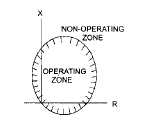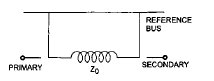Courses

# Test: Power Systems - 9

## 25 Questions MCQ Test Electrical Engineering SSC JE (Technical) | Test: Power Systems - 9

Description
This mock test of Test: Power Systems - 9 for Electrical Engineering (EE) helps you for every Electrical Engineering (EE) entrance exam. This contains 25 Multiple Choice Questions for Electrical Engineering (EE) Test: Power Systems - 9 (mcq) to study with solutions a complete question bank. The solved questions answers in this Test: Power Systems - 9 quiz give you a good mix of easy questions and tough questions. Electrical Engineering (EE) students definitely take this Test: Power Systems - 9 exercise for a better result in the exam. You can find other Test: Power Systems - 9 extra questions, long questions & short questions for Electrical Engineering (EE) on EduRev as well by searching above.
QUESTION: 1

### An impedance relay can be used for

Solution:

Explanation : The impedance relay is better suited for phase-fault relaying for lines of moderate length than for either very short or very long lines. Arcs affect an impedance relay more than a reactance relay but less than a mho relay.

QUESTION: 2

Solution:
QUESTION: 3

### The operating characteristic of a distance relay in the R–X plane is shown in the figure. It represent operating characteristic of aSolution:
QUESTION: 4

Which of the following relays has inherent directional characteristics?

Solution:
QUESTION: 5

Differential relays are used for protection of

Solution:
QUESTION: 6

A three-phase transformer having zero sequence impedance of Z0 has the zero-sequence network as shown in the figure. The connection of its windings areSolution:
QUESTION: 7

In Merz-Price percentage differential protection of a D- Y transformer, the CT secondaries connection in the primary and secondary winding of the transformer would be in the form of

Solution:
QUESTION: 8

Zero-sequence impedance of the transmission line is

Solution:
QUESTION: 9

The most commonly used method for the protection of 3–phase feeder is

Solution:
QUESTION: 10

For protection of parallel feeders fed from one end, the relays required are

Solution:
QUESTION: 11

An overhead transmission line is provided with earth wire for protection against.

Solution:
QUESTION: 12

Conductivity of aluminium, as compared to copper is

Solution:
QUESTION: 13

The type of insulators used on a 132 KV line are

Solution:
QUESTION: 14

Porcelain insulators are glazed to

Solution:
QUESTION: 15

Series capacitors are used to

Solution:
QUESTION: 16

The protection from negative sequence currents is provided for

Solution:
QUESTION: 17

A system which is more prone to faults is

Solution:
QUESTION: 18

PVC cables are usually used for

Solution:
QUESTION: 19

Solution:
QUESTION: 20

The resistance ofearth should be

Solution:
QUESTION: 21

Transmission of power by ac cables is impossible beyond

Solution:
QUESTION: 22

For a high speed circuit breaker the total clearing time is around

Solution:
QUESTION: 23

The RRRV depends upon the

Solution:
QUESTION: 24

What is te preferred type of CB to be installed in extra high voltage ac system ?

Solution:
QUESTION: 25

The primary function of a fuse is to

Solution: